# How to Use the Excel SEQUENCE FunctionClaudia Buckley#### Join the Excel conversation on Slack

Ask a question or join the conversation for all things Excel on our Slack channel.

The SEQUENCE function in Excel is a dynamic array function that was introduced in Excel 365. It allows you to easily create arrays of any size and shape, and populate them with sequential numerical values based on a variety of criteria.

## Syntax

The syntax of the SEQUENCE function is:

=SEQUENCE(rows, [columns], [start], [step])

where:

• rows: the number of rows you want to generate.
• columns (optional): the number of columns you want to generate. If omitted, it defaults to 1.
• start (optional): the first number in the sequence. If omitted, it defaults to 1.
• step (optional): the increment between each number in the sequence. If omitted, it defaults to 1.

In this article, we will explore some common uses of the SEQUENCE function in Excel.

## How to generate a sequence of numbers in Excel

The most basic use of the SEQUENCE function is to generate a sequence of numbers. For example, to generate a sequence of numbers from 1 to 10, you can use the formula:

``=SEQUENCE(10)``

The optional arguments can be omitted in this case since the default parameters align with our desired result. This will generate a column of 10 numbers, starting from 1 and incrementing by 1.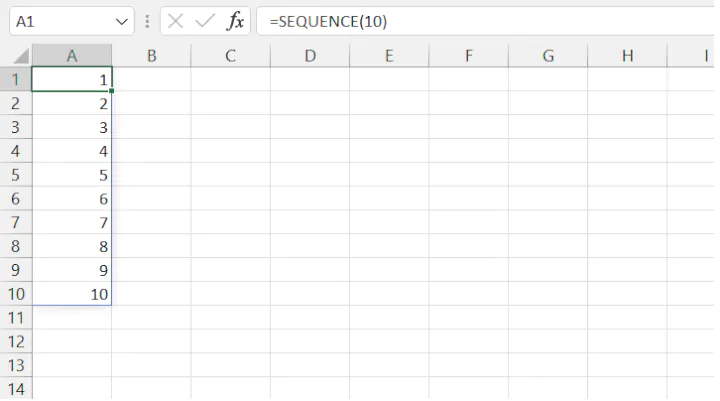This single input will create a "spill" of values into the neighboring rows, provided they are empty. To generate a row of numbers, you can use the following formula:

``=SEQUENCE(1,10)``

This will generate a row of 10 numbers, starting from 1 and incrementing by 1.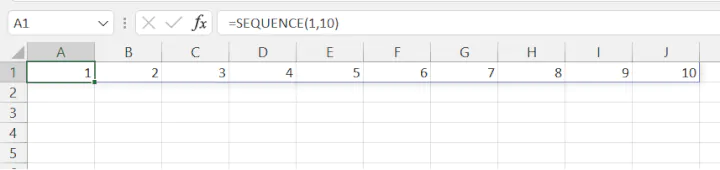You can also change the starting number and the increment between each number. For example, to generate a sequence of even numbers from 2 to 20, you can use the formula:

``=SEQUENCE(10,1,2,2)``

This will generate a column of 10 even numbers, starting from 2 and incrementing by 2.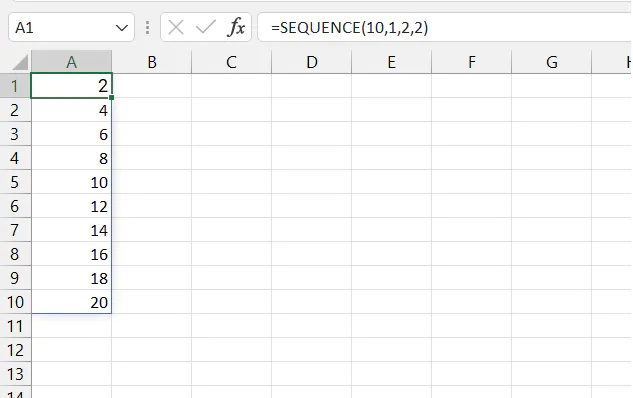## Generate a sequence of dates in Excel

The SEQUENCE function can also be used to generate a sequence of dates. For example, to generate a column of dates for the month of January 2022, you can use the formula:

``=SEQUENCE(31,1,DATE(2022,1,1),1)``

This will generate a column of 31 dates, starting from January 1st, 2022, and incrementing by 1 day.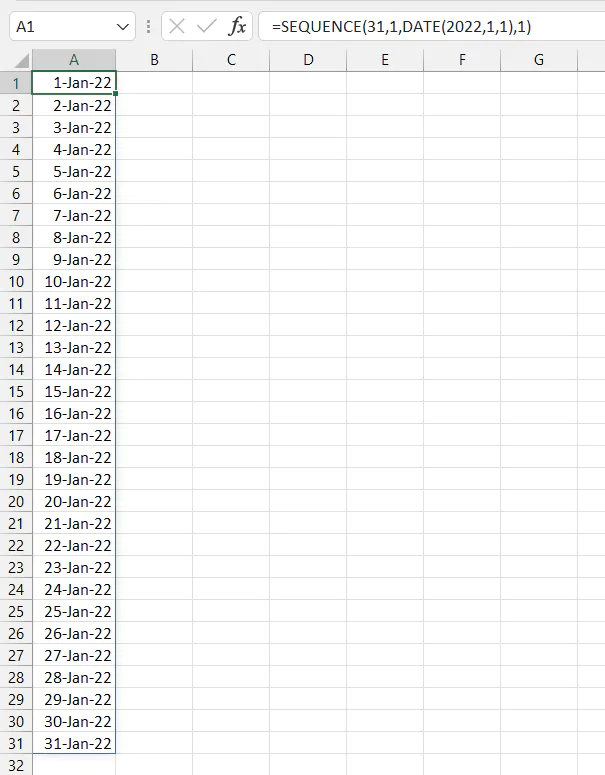Remember that in order to get your dates in Excel to display as dates, you will need to change your number format from General to Date. Otherwise, Excel may return serial numbers which do not appear to be dates at all!

The TRANSPOSE function converts a vertical range of cells to a horizontal range, or vice versa. You can generate a row of dates by adding the TRANSPOSE function.

``=TRANSPOSE(SEQUENCE(31,1,DATE(2022,1,1),1))``

This will generate a row of 31 dates, starting from January 1st, 2022 and incrementing by 1 day.

## Generate a grid of values in Excel

The SEQUENCE function can also be used to generate a grid of values. For example, to generate a 3x3 grid of sequential numbers beginning with the number 1, you can use the formula:

``=SEQUENCE(3,3,1,1)``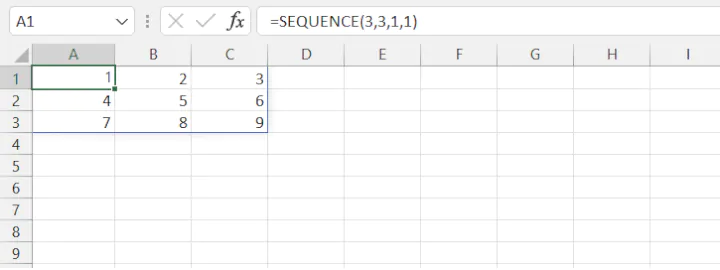These are just a few basic examples of how SEQUENCE can be used. However, its capabilities don't end here. For example, by nesting (combining) SEQUENCE with other formulas, you can generate a sequence of random numbers or dates, like in this example.

## Wrapping up

In conclusion, the SEQUENCE function in Excel is a powerful tool that can help you generate sequences of numbers, dates, or text strings quickly and easily. With its flexible syntax and ability to generate arrays of any size and shape, SEQUENCE is a useful function for a variety of tasks, from simple number generation to complex data analysis.

By using the SEQUENCE function in combination with other Excel functions, you can create dynamic, customizable spreadsheets that can adapt to changing data and analysis needs. So next time you need to generate a sequence of numbers, dates, or text strings in Excel, remember to try the SEQUENCE function and see how it can help you streamline your work and improve your improve your productivity.

## Next steps

To learn how to use other dynamic array functions in Excel, check out our Excel 365 - Basic and Advanced course.

Become a certified Excel ninja with GoSkills bite-sized courses#### Join the Excel conversation on Slack

Ask a question or join the conversation for all things Excel on our Slack channel.Claudia Buckley

Claudia is a project manager and business skills instructor at GoSkills. In her spare time, she reads mystery novels and does genealogy research.